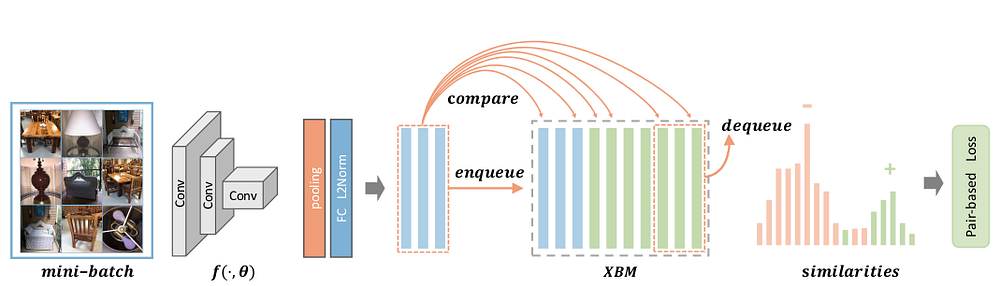# Cross Batch Model — XBM

Original article can be found here (source): Deep Learning on Medium# INTRODUCTION

Deep metric learning (DML) aims to learn an embedding space where instances from the same class are encouraged to be closer than those from different classes.

A family of DML approaches are known as pair-based, whose objectives can be defined in terms of pair-wise similarities within a mini-batch, such as contrastive loss, triplet loss, lifted-structure loss, n-pairs loss, multi-similarity (MS) loss and etc.

The performance of pair-based methods heavily relies on their capability of mining informative negative pairs. Negative pairs are pairs of data points that belong to different classes.

Negative pairs can be easily increased by increasing the size of mini-batch, however, this solution has a limitation, that the mini-batch size is limited by the GPU memory and computational cost.

This paper proposes a solution for increasing the hard negative without much computational overhead.

# LOSS CALCULATION IN PAIR BASED DML

The loss has 2 parts :

1. Sum of similarity in negative pairs

Here Sij is the similarity between the anchor and a negative query

2.Sum of similarity in positive pairs

Here Sij is the similarity between the anchor and a positive query

We have to decrease the similarity of negative pairs and increase it for the positive pairs.

Hence the total loss becomes:

As loss will decrease, the similarity of negative pairs will decrease and that of positive pairs will increase.

From this equation, we can conclude that increasing the negative pairs will give more information to the model and the model will train more efficiently.

# SLOW DRIFT

The embeddings of past mini-batches are usually considered out-of-date because the model parameters are changing throughout the training process. And hence, old embeddings should be discarded. But this is not always true.

With a certain number of training iterations, the embeddings of instances can drift very slowly,resulting in marginal differences between the features computed at different training iterations.

This phenomenon is known as Slow Drift.

Hence we can say that if slow Drift of embeddings is occurring then some previous embeddings of mini-batches are useful as not much is learned by the model.

# CROSS BATCH MODEL (XBM)

XBM provides plentiful hard negative pairs by directly connecting each anchor in the current mini-batch with the embeddings from recent mini-batches.

As the feature drift is relatively large at the early epochs, the neural networks are warmed up allowing the model to reach a certain local optimal field where the embeddings become more stable. Then we initialize the memory Batch by computing the features of a set of randomly sampled training images with the warm-up model.

A queue Data structure is used to maintain the Memory Batch. At each iteration, the enqueue of the latest embeddings and dequeue of the earliest embeddings is done. Thus memory batch is updated with embeddings of the current mini-batch directly, without any additional computation.

The Memory batch constructed consists of a good amount of negative pairs and hence helps the network to learn faster.

# ALGORITHM

`train network f conventionally with K epochsinitialize XBM as queue Mfor x, y in loader: # x: data, y: labels anchors = f.forward(x)# memory update enqueue(M, (anchors.detach(), y)) dequeue(M)# compare anchors with M sim = torch.matmul(anchors.transpose(), M.feats) loss = pair_based_loss(sim, y, M.labels) loss.backward() optimizer.step()`

# RESULTS

The model was tested with SOP, In-shop and Vehicle ID Data sets